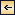Statics and Kinematics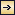### The Fields of Gravity, Pressure, and Mass

Level Surfaces. Coordinate surfaces of equal geometric depth below the ideal sea surface are useful when considering geometrical features, but in problems of statics or dynamics that involve consideration of the acting forces, they are not always satisfactory. Because the gravitational force represents one of the most important of the acting forces, it is convenient to use as coordinate surfaces the level surfaces, defined as surfaces that are everywhere normal to the force of gravity. It will presently be shown that these surfaces do not coincide with surfaces of equal geometric depth.

It follows from the definition of level surfaces that, if no forces other than gravitational are acting, a mass can be moved along a level surface without expenditure of work and that the amount of work expended or gained by moving a unit mass from one surface to another is independent of the path taken.

The amount of work, W, required for moving a unit mass a distance, h, along the plumb line is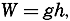[Equation]

403
where g is the acceleration of gravity. Work per unit mass has dimensions L2T−2, and the numerical value depends therefore only on the units used for length and time. When the length is measured in meters and the time in seconds, the unit of work per unit mass is called a dynamic decimeter (Bjerknes and different collaborators, 1910).

In the following, the sea surface will be considered a level surface. The work required or gained in moving a unit mass from sea level to a point above or below sea level is called the gravity potential, and in the m.t.s. system the unit of gravity potential is thus one dynamic decimeter.

The practical unit of the gravity potential is the dynamic meter, for which the symbol D is used. When dealing with the sea the vertical axis is taken as positive downward. The geopotential of a level surface at the geometrical depth, z, is therefore, in dynamic meters,[Equation]
The geometrical depth in meters of a given level surface, on the other hand, is[Equation]

The acceleration of gravity varies with latitude and depth, and the geometrical distance between standard level surfaces therefore varies with the coordinates. At the North Pole the geometrical depth of the 1000-dynamic-meter surface is 1017.0 m, but at the Equator the depth is 1022.3 m, because g is greater at the Poles than at the Equator. Thus, level surfaces and surfaces of equal geometric depth do not coincide. Level surfaces slope relative to the surfaces of equal geometric depth, and therefore a component of the acceleration of gravity acts along surfaces of equal geometrical depth.

The topography of the sea bottom is represented by means of isobaths—that is, lines of equal geometrical depth—but it could be presented equally well by means of lines of equal geopotential. The contour lines would then represent the lines of intersection between the level surfaces and the irregular surface of the bottom. These contours would no longer be at equal geometric distances, and hence would differ from the usual topographic chart, but their characteristics would be that the amount of work needed for moving a given mass from one contour to another would be constant. They would also represent the new coast lines if the sea level were lowered without alterations of the topographic features of the bottom, provided the new sea level would assume perfect hydrostatic equilibrium and adjust itself normal to the gravitational force.

Any scalar field can similarly be represented by means of a series of topographic charts of equiscalar surfaces in which the contour lines

404
represent the lines of intersection of the level surfaces with the equiscalar surface. Charts of this character will be called geopotential topographic charts, or charts of geopotential topography, in contrast to topographic charts in which the contour lines represent the lines along which the depth of the surface under consideration is constant.

The Field of Gravity. The fact that gravity is the resultant of two forces, the attraction of the earth and the centrifugal force due to the earth's rotation, need not be considered, and it is sufficient to define gravity as the force that is derived empirically by pendulum observations. Furthermore, it is not necessary to take into account the minor irregular variations of gravity that detailed surveys reveal, but it is enough to make use of the “normal” value, in meters per second per second, which at sea level can be represented as a function of the latitude, ϕ, by Helmert's formula: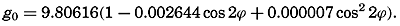[Equation]
Thus, the normal value at the poles is 9.83205, and at the Equator it is 9.78027. The normal value of g increases with depth, according to the formula[Equation]
From formula (XII, 1) one obtains in dynamic meters the geopotential that corresponds to a given depth, z: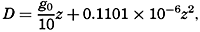[Equation]
or from (XII, 2) the depth corresponding to a given value of D: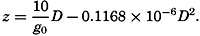[Equation]
In the first approximation,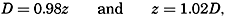[Equation]
meaning that the numbers which represent the depth in meters deviate only by about 2 per cent from the numbers which represent the geopotential in dynamic meters. Extensive use will be made of this numerical agreement between the two units, but it must always be borne in mind that a dynamic meter is a measure of work per unit mass, and not a measure of length. The conversion factors, 0.98 and 1.02, are therefore not pure numbers, but the former has the dimensions LT−2 and the latter has the dimensions L−1T2.

The field of gravity can be completely described by means of a set of equipotential surfaces corresponding to standard intervals of the gravity potential. These are at equal distances if the geopotential is

405
used as the vertical coordinate, but, if geometric depth is used, the distance between the equipotential surfaces varies. If the field is represented by equipotential surfaces at intervals of one dynamic decimeter, it follows from the definition of these surfaces that the numerical value of the acceleration of gravity is the reciprocal of the geometric thickness in meters of the unit sheets.

The Field of Pressure. The distribution of pressure in the sea can be determined by means of the equation of static equilibrium: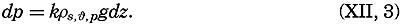[Equation]
Here, k is a numerical factor that depends on the units used, and ρs, ϕ p is the density of the water (p. 56).

The hydrostatic equation will be discussed further in connection with the equations of motion (p. 440). At this time it is enough to emphasize that, as far as conditions in the ocean are concerned, the equation, for all practical purposes, is exact.

Introducing the geopotential expressed in dynamic meters as the vertical coordinate, one has 10dD = gdz. When the pressure is measured in decibars (defined by 1 bar = 106 dynes per square centimeter), the factor k becomes equal to 1/10, and equation (XII, 3) is reduced to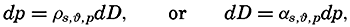[Equation]
where α s, ϕ, p is the specific volume.

Because ρs, ϕ, pand αs, ϕ, p differ little from unity, a difference in pressure is expressed in decibars by nearly the same number that expresses the difference in geopotential in dynamic meters, or the difference in geometric depth in meters. Approximately,[Equation]

The pressure field can be completely described by means of a system of isobaric surfaces. Using the geopotential as the vertical coordinate, one can present the pressure distribution by a series of charts showing isobars at standard level surfaces or by a series of charts showing the geopotential topography of standard isobaric surfaces. In meteorology, the former manner of representation is generally used on weather maps, in which the pressure distribution at sea level is represented by isobars. In oceanography, on the other hand, it has been found practical to represent the geopotential topography of isobaric surfaces.

The pressure gradient is defined by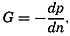[Equation]
where n is directed normal to the isobaric surfaces (p. 156). The pressure
406
gradient has the character of a force per unit volume, because pressure has the dimensions of a force per unit area. The dimension of a pressure is ML−1 T−2 = MLT−2 × L−2, and the dimension of a pressure gradient is ML−2 T−2 = MLT−2 × L−3. Multiplying the pressure gradient by the specific volume, M−1L3, one obtains a force per unit mass of dimensions LT−2.

The pressure gradient has two principal components: the vertical, directed normal to the level surfaces, and the horizontal, directed parallel to the level surfaces. When static equilibrium exists, the vertical component, expressed as force per unit mass, is balanced by the acceleration of gravity. This is the statement which is expressed mathematically by means of the equation of hydrostatic equilibrium. In a resting system the horizontal component of the pressure gradient is not balanced by any other force, and therefore the existence of a horizontal pressure gradient indicates that the system is not at rest or cannot remain at rest. The horizontal pressure gradients, therefore, although extremely small, are all-important to the state of motion, whereas the vertical are insignificant in this respect.

It is evident that no motion due to pressure distribution exists or can develop if the isobaric surfaces coincide with level surfaces. In such a state of perfect hydrostatic equilibrium the horizontal pressure gradient vanishes. Such a state would be present if the atmospheric pressure, acting on the sea surface, were constant, if the sea surface coincided with the ideal sea level and if the density of the water depended on pressure only. None of these conditions is fulfilled. The isobaric surfaces are generally inclined relative to the level surfaces, and horizontal pressure gradients are present, forming a field of internal force.

This field of force can also be defined by considering the slopes of isobaric surfaces instead of the horizontal pressure gradients. By definition the pressure gradient along an isobaric surface is zero, but, if this surface does not coincide with a level surface, a component of the acceleration of gravity acts along the isobaric surface and will tend to set the water in motion, or must be balanced by other forces if a steady state of motion is reached. The internal field of force can therefore be represented also by means of the component of the acceleration of gravity along isobaric surfaces (p. 440).

Regardless of the definition of the field of force that is associated with the pressure distribution, for a complete description of this field one must know the absolute isobars at level surfaces or the absolute geopotential contour lines of isobaric surfaces. These demands cannot possibly be met. One reason is that measurements of geopotential distances of isobaric surfaces must be made from the actual sea surface, the topography of which is unknown. It will be shown that all one can do is to determine the pressure field that would be present if the pressure

407
distribution depended only upon the distribution of mass in the sea. This part of the total pressure field will be called the relative field of pressure, but it cannot be too strongly emphasized that the total field of pressure is composed of this relative field and, in addition, a field that is maintained by external forces such as atmospheric pressure and wind.

In order to illustrate this point a fresh-water lake will be considered which is so small that horizontal differences in atmospheric pressure can be disregarded and the acceleration of gravity can be considered constant. Let it first be assumed that the water is homogeneous, meaning that the density is independent of the coordinates. In this case, the distance between any two isobaric surfaces is expressed by the equation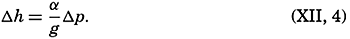[Equation]

This equation simply states that the geometrical distance between isobaric surfaces is constant, and it defines completely the internal field of pressure. The total field of pressure depends, however, upon the configuration of the free surface of the lake. If no wind blows and if no stress is thus exerted on the free surface of the lake, perfect hydrostatic equilibrium exists, the free surface is a level surface, and, similarly, all other isobaric surfaces coincide with level surfaces. On the other hand, if a wind blows across the lake, the equilibrium will be disturbed, the water level will be lowered at one end of the lake, and water will be piled up against the other end. The free surface will still be an isobaric surface, but it will now be inclined relative to a level surface. The relative field of pressure, however, will remain unaltered as represented by equation (XII, 4), meaning that all other isobaric surfaces will have the same geometric shape as that of the free surface.

One might continue and introduce a number of layers of different density, and one would find that the same reasoning would be applicable. The method is therefore also applicable when one deals with a liquid within which the density changes continually with depth. By means of observations of the density at different depths, one can derive the relative field of pressure and can represent this by means of the topography of the isobaric surfaces relative to some arbitrarily or purposely selected isobaric surface. The relative field of force can be derived from the slopes of the isobaric surface relative to the selected reference surface, but, in order to find the absolute field of pressure and the corresponding absolute field of force, it is necessary to determine the absolute shape of one isobaric surface.

These considerations have been set forth in great detail because it is essential to be fully aware of the difference between the absolute field of pressure and the relative field of pressure, and to know what types of data are needed in order to determine each of these fields.

408

The Field of Mass. The field of mass in the ocean is generally described by means of the specific volume as expressed by (p. 57)[Equation]
The field of the specific volume can be considered as composed of two fields, the field of α35, 0,p and the field of δ.

The former field is of a simple character. The surfaces of α35,0,p coincide with the isobaric surfaces, the deviations of which from level surfaces are so small that for practical purposes the surfaces of α35,0,p can be considered as coinciding with level surfaces or with surfaces of equal geometric depth. The field of α35,0,p can therefore be fully described by means of tables giving α35,0,p as a function of pressure and giving the average relationships between pressure, geopotential and geometric depths. Since this field can be considered a constant one, the field of mass is completely described by means of the anomaly of the specific volume, δ, the determination of which was discussed on p. 58.

The field of mass can be represented by means of the topography of anomaly surfaces or by means of horizontal charts or vertical sections in which curves of δ = constant are entered. The latter method is the most common. It should always be borne in mind, however, that the specific volume in situ is equal to the sum of the standard specific volume, α35,0,p at the pressure in situ and the anomaly, δ.

The Relative Field of Pressure. It is impossible to determine the relative field of pressure in the sea by direct observations, using some type of pressure gauge, because an error of only 0.1 m in the depth of a pressure gauge below the sea surface would introduce errors greater than the horizontal differences that should be established. If the field of mass is known, however, the internal field of pressure can be determined from the equation of static equilibrium in one of the forms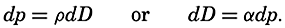[Equation]
In oceanography the latter form has been found to be the more practical, but all reasoning applies equally well to results deduced from the former.

Integration of the latter form gives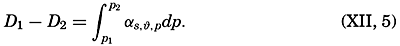[Equation]
Because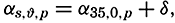[Equation]
one can write[Equation]
where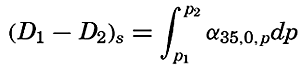[Equation]
and is called the standard geopotential distance between the isobaric
409
surfaces p1 and p2, and where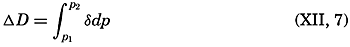[Equation]
is called the anomaly of the geopotential distance between the isobaric surfaces p1 and p2, or, abbreviated, the geopotential anomaly.

Equation (XII, 6) can be interpreted as expressing that the relative field of pressure is composed of two fields: the standard field and the field of anomalies. The standard field can be determined once and for all, because the standard geopotential distance between isobaric surfaces represents the distance if the salinity of the sea water is constant at 35 ‰ and the temperature is constant at 0°C. The standard geopotential distance decreases with increasing pressure, because the specific volume decreases (density increases) with pressure, as is evident from table 7H in Bjerknes (1910), according to which the standard geopotential distance between the isobaric surfaces 0 and 100 decibars is 97.242 dynamic meters, whereas the corresponding distance between the 5000-and 5100-decibar surfaces is 95.153 dynamic meters.

The standard geopotential distance between any two standard isobaric surfaces is, on the other hand, independent of latitude, but the geometric distance between isobaric surfaces varies with latitude because g varies.

Because in the standard field all isobaric surfaces are parallel relative to each other, this standard field lacks a relative field of horizontal force. The relative field of force, which is associated with the distribution of mass, is completely described by the field of the geopotential anomalies. It follows that a chart showing the topography of one isobaric surface relative to another by means of the geopotential anomalies is equivalent to a chart showing the actual geopotential topography of one isobaric surface relative to another. The practical determination of the relative field of pressure is therefore reduced to computation and representation of the geopotential anomalies, but the absolute pressure field can be found only if one can determine independently the absolute topography of one isobaric surface.

In order to evaluate equation (XII, 7), it is necessary to know the anomaly, δ, as a function of absolute pressure. The anomaly is computed from observations of temperature and salinity, but oceanographic observations give information about the temperature and the salinity at known geometrical depths below the actual sea surface, and not at known pressures. This difficulty can fortunately be overcome by means of an artificial substitution, because at any given depth the numerical value of the absolute pressure expressed in decibars is nearly the same as the numerical value of the depth expressed in meters, as is evident from the following corresponding values:

410
 Standard sea pressure (decibars) 1000 2000 3000 4000 5000 6000 Approximate geometric depth (m) 990 1975 2956 3933 4906 5875

Thus, the numerical values of geometric depth deviate only 1 or 2 percent from the numerical values of the standard pressure at that depth. This agreement is not accidental, but has been brought about by the selection of the practical unit of pressure, the decibar.

It follows that the temperature at a pressure of 1000 decibars is nearly equal to the temperature at a geometric depth of 990 m, or the temperature at the pressure of 6000 decibars is nearly equal to the temperature at a depth of 5875 m. The vertical temperature gradients in the ocean are small, especially at great depths, and therefore no serious error is introduced if, instead of using the temperature at 990 m when computing δ, one makes use of the temperature at 1000 m, and so on. The difference between anomalies for neighboring stations will be even less affected by this procedure, because within a limited area the vertical temperature gradients will be similar. The introduced error will be nearly the same at both stations, and the difference will be an error of absolutely negligible amount. In practice one can therefore consider the numbers that represent the geometric depth in meters as representing absolute pressure in decibars. If the depth in meters at which either directly observed or interpolated values of temperature and salinity are available is interpreted as representing pressure in decibars, one can compute, by means of the tables in the appendix, the anomaly of specific volume at the given pressure. By multiplying the average anomaly of specific volume between two pressures by the difference in pressure in decibars (which is considered equal to the difference in depth in meters), one obtains the geopotential anomaly of the isobaric sheet in question expressed in dynamic meters. By adding these geopotential anomalies, one can find the corresponding anomaly between any two given pressures. An example of a complete computation is given in table 61.

Certain simple relationships between the field of pressure and the field of mass can be derived by means of the equations for equiscalar surfaces (p. 155) and the hydrostatic equation. In a vertical profile the isobars and the isopycnals are defined by[Equation]
The inclinations of the isobars and isopycnals are therefore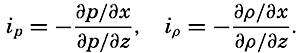[Equation]
By means of the hydrostatic equation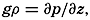[Equation]

411
 Meters or decibars Temp. (°C) Salinity (‰) σt 105Δs,ϕ 105δs,p 105δϕ,p 105δ ΔD ΔD(dynamic meter) 0 14.22 33.25 24.81 315.0 315 10 13.72 .24 .91 305.5 0.3 306 .0310 .0310 25 .71 .24 .91 305.5 0.7 306 .0459 .0769 50 .35 .30 25.03 294.2 −0.1 1.3 296 .0752 .1521 75 9.96 .57 .86 215.2 −0.2 1.6 217 .0641 .2162 100 .38 .84 26.17 185.7 −0.3 2.0 187 .0505 .2667 150 8.82 .98 .37 166.5 −0.3 2.9 169 .0890 .3557 200 .48 34.09 .51 153.5 −0.3 3.7 157 .0815 .4372 250 .30 .16 .59 145.9 −0.4 4.6 150 .0768 .5140 300 7.87 .20 .69 136.4 −0.5 5.2 141 .0728 .5868 400 .07 .20 .80 125.9 −0.6 6.4 132 .1365 .7233 500 6.14 .26 .97 109.8 −0.7 7.2 116 .1240 .8473 600 5.51 .35 27.12 95.6 −0.8 7.9 103 .1095 .9568 800 4.65 .42 .28 80.4 −1.0 8.9 88 .1910 1.1478 1000 3.99 .44 .36 72.9 −1.2 9.8 82 1700 1.3178 1200 .52 .52 .48 61.5 −1.4 10.3 70 .1520 1.470 1400 .07 .54 .54 55.8 −1.0 10.8 66 .1360 1.606 1600 2.69 .56 .59 51.1 −1.0 10.9 61 .1270 1.733 1800 .37 .59 .64 46.3 −1.0 10.8 56 .1170 1.850 2000 .13 .64 .69 41.6 −1.1 10.6 51 .1070 1.957 3000 1.62 .68 .76 35.0 −1.4 11.7 45 .4800 2.437 4000 .50 .70 .81 30.2 −1.7 14.1 43 .4400 2.877

one obtains[Equation]
From the two latter equations it follows that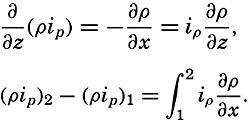[Equation]
If the density distribution is represented by means of unit sheets (p. 156), the integral on the right-hand side can be evaluated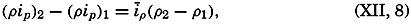[Equation]
where īp means the average inclination of unit sheets. The isobaric surface p1 lies above the surface p2, because the vertical axis is positive downward. The inclination of the upper isobaric surface relative to the lower, ip1−p2, therefore, when an average value of the density is introduced,
412
is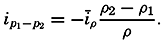[Equation]
Using the specific volume anomaly and making the specific volume equal to unity, one obtains approximately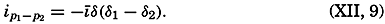[Equation]
Because δ decreases with depth, δ1 - δ2 is positive, and the inclination of one isobaric surface relative to another is of opposite sign to the inclination of the δ surfaces (p. 449). This rule permits a rapid estimate of the relative inclinations of isobaric surface in a section in which the field of mass has been represented by δ curves.

Profiles of isobaric surfaces based on the data from a series of stations in a section must evidently be in agreement with the inclination of the δ curves, as shown in a section and based on the same data, but this obvious rule often receives little or no attention.

Relative Geopotential Topography of Isobaric Surfaces. If simultaneous observations of the vertical distribution of temperature and salinity were available from a number of oceanographic stations within a given area, the relative pressure distribution at the time of the observations could be represented by a series of charts showing the geopotential topography of standard isobaric surfaces relative to one arbitrarily or purposely selected reference surface. From the preceding it is evident that these topographies are completely represented by means of the geopotential anomalies.

In practice, simultaneous observations are not available, but in many instances it is permissible to assume that the time changes of the pressure distribution are so small that observations taken within a given period may be considered simultaneous. The smaller the area, the shorter must be the time interval within which the observations are made. Figs. 110, p. 454 and 204, p. 726, represent examples of geopotential topographies. The conclusions as to currents which can be based on such charts will be considered later.

Charts of geopotential topographies can be prepared in two different ways. By the common method, the anomalies of a given surface relative to the selected reference surface are plotted on a chart and isolines are drawn, following the general rules for presenting scalar quantities. In this manner, relative topographies of a series of isobaric surfaces can be prepared, but the method has the disadvantage that each topography is prepared separately.

By the other method a series of charts of relative topographies is prepared stepwise, taking advantage of the fact that the anomaly of geopotential thickness of an isobaric sheet is proportional to the average

413
specific volume anomaly,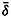[Equation], in the isobaric sheet. Thus, if the thickness of the isobaric sheet is 100 decibars, δDp1p1-100 = 100δ. Consequently, curves of 100δ represent the topography of the surface p = p1 − 100 relative to the surface p = p1, and one can proceed as follows: The topography of the surface ps − 200, where ps is the selected reference surface, is constructed by means of curves of equal values of the specific volume anomaly in the sheet ps to ps − 100. The topography of the surface ps − 200 relative to the surface ps − 100 is constructed by means of the specific volume anomalies in the sheet ps − 100 to ps − 200, and by graphical addition of these two charts (V. Bjerknes and collaborators, 1911) the topography of the surface ps − 200 relative to the surface ps is found. This process can be repeated, and by successive constructions of charts and specific volume anomalies and by graphical additions, the entire fields of mass and pressure can be represented.

This method is widely used in meteorology, but is not commonly employed in oceanography because, for the most part, the different systems of curves are so nearly parallel to each other that graphical addition is cumbersome. The method is occasionally useful, however, and has the advantage of showing clearly the relationship between the distribution of mass and the distribution of pressure. It especially brings out the geometrical feature that the isohypses of the isobaric surfaces retain their form when passing from one isobaric surface to another only if the anomaly curves are of the same form as the isohypses. This characteristic of the field is of great importance to the dynamics of the system.

Character of the Total Field of Pressure. From the above discussion it is evident that, in the absence of a relative field of pressure, isosteric and isobaric surfaces must coincide. Therefore, if for some reason one isobaric surface, say the free surface, deviates from a level surface, then all isobaric and isosteric surfaces must deviate in a similar manner. Assume that one isobaric surface in the disturbed condition lies at a distance Δh cm below the position in undisturbed conditions. Then all other isobaric surfaces along the same vertical are also displaced the distance Δh from their undisturbed position. The distance Δh is positive downward because the positive z axis points downward. Call the pressure at a given depth at undisturbed conditions p0. Then the pressure at disturbed conditions is pt = p0 − Δp, where Δp = gρΔh and where the displacement Δh can be considered as being due to a deficit or an excess of mass in the water column under consideration.

The above considerations are equally valid if a relative field of pressure exists. The absolute distribution of pressure can always be completely determined from the equation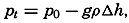[Equation]

414
and would therefore be fully known if one could determine Δh, the vertical displacement of the isobaric surfaces due to excess or deficit of mass in the column under consideration. An added horizontal field of force is present when this vertical displacement varies from one locality to another, in which case the absolute isobaric surfaces slope in relation to the isobaric surfaces of the relative field. The added field can be called the slope field, and this analysis thus leads to the result that the total field of pressure is composed of the internal field and the slope field. This distinction is helpful when discussing the character of the currents.Statics and Kinematics